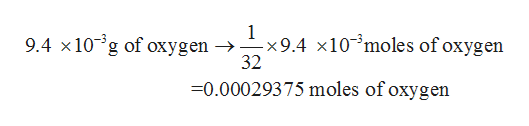Seawater at 15°C contains 9.4 mg/L of molecular oxygen. What molarity is this?

Question

Seawater at 15°C contains 9.4 mg/L of molecular oxygen. What molarity is this?

Step 1

The number of moles of a given chemical species is defined as the ratio of mass to the molar mass of the substance. The mathematical formula is as shown below:

Step 2

The term molarity or concentration for a given solution is defined as the ratio of number of moles to the volume of the solution (in liters). Its SI units are moles/liter.

Step 3

From the mole concept, it is well known that 1 mole of oxygen contains 32 g of oxygen.

This means that 32 ...help_outlineImage Transcriptionclose1 -x9.4 x10 moles of oxygen 32 9.4 x10 g of oxygen -> X 0.00029375 moles of oxygen fullscreen

Want to see the full answer?

See Solution

Want to see this answer and more?

Our solutions are written by experts, many with advanced degrees, and available 24/7

See Solution
Tagged in

Inorganic Chemistry